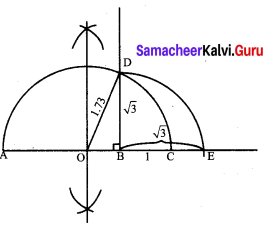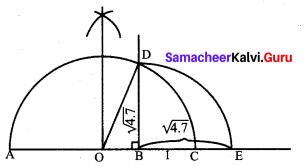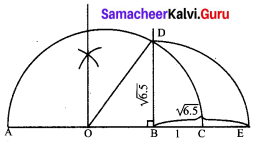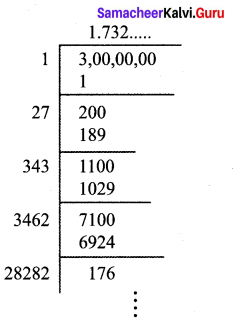You can Download Samacheer Kalvi 9th Maths Book Solutions Guide Pdf, Tamilnadu State Board help you to revise the complete Syllabus and score more marks in your examinations.

## Tamilnadu Samacheer Kalvi 9th Maths Solutions Chapter 2 Real Numbers Ex 2.3

Question 1.
Represent the following irrational numbers on the number line.
(i) $$\sqrt { 3}$$
(ii) $$\sqrt { 4.7 }$$
(iii) $$\sqrt { 6.5 }$$
Solution:
(i) $$\sqrt { 3}$$(i) Draw a line and mark a point A on it.
(ii) Mark a point B such that AB = 3 cm.
(iii) Mark a point C on this line such that BC = 1 unit.
(iv) Find the midpoint of AC by drawing perpendicular bisector of AC and let it be O.
(v) With O as center and OC = OA as radius, draw a semicircle.
(vi) Draw a line BD, which is perpendicular to AB at B.
(vii) Now BD = $$\sqrt { 3}$$, which can be marked in the number line as the value of BE = BD = $$\sqrt { 3}$$

(ii) $$\sqrt { 4.7 }$$(i) Draw a line and mark a point A on it.
(ii) Mark a point B such that AB = 4.7 cm.
(iii) Mark a point C on this line such that BC = 1 unit.
(iv) Find the midpoint of AC by drawing perpendicular bisector of AC and let it be O.
(v) With O as center and OC = OA as radius, draw a semicircle.
(vi) Draw a line BD, which is perpendicular to AB at B.
(vii) Now BD = $$\sqrt { 4.7 }$$, which can be marked in the number line as the value of BE = BD = $$\sqrt { 4.7 }$$

(iii) $$\sqrt { 6.5 }$$(i) Draw a line and mark a point A on it.
(ii) Mark a point B such that AB = 6.5 cm.
(iii) Mark a point C on this line such that BC = 1 unit.
(iv) Find the midpoint of AC by drawing perpendicular bisector of AC and let it be O.
(v) With O as center and OC = OA as radius, draw a semicircle.
(vi) Draw a line BD, which is perpendicular to AB at B.
(vii) Now BD = $$\sqrt { 6.5 }$$, which can be marked in the number line as the value of BE = BD = $$\sqrt { 6.5 }$$.

Question 2.
Find any two irrational numbers between
(i) 0.3010011000111…. and 0.3020020002….
(ii) $$\frac { 6 }{ 7 }$$ and $$\frac { 12 }{ 13 }$$
(iii) $$\sqrt { 2}$$ and $$\sqrt { 3}$$
Solution:
(i) 0.3010011000111…. and 0.3020020002….
Two irrational numbers 0.301202200222 …., 0.301303300333…..

(ii) $$\frac { 6 }{ 7 }$$ and $$\frac { 12 }{ 13 }$$
$$\frac { 6 }{ 7 }$$ = 0.857142…
$$\frac { 12 }{ 13 }$$ = 0.923076
Two irrational numbers between 0.8616611666111……, 0.8717711777111…..

(iii) $$\sqrt { 2}$$ and $$\sqrt { 3}$$$$\sqrt { 2 }$$= 1.414……$$\sqrt { 3}$$ = 1.732….
∴ Two irrational numbers between 1.5155…., 1.6166…….Question 3.
Find any two rational numbers between 2.2360679….. and 2.236505500….
Solution:
Any two rational numbers are 2.2362, 2.2363